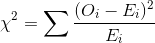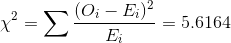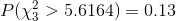##### Question

In: Statistics and Probability

# The value of a college degree is greater than it has been in nearly half a...

The value of a college degree is greater than it has been in nearly half a century, a least when compared to the prospect of not getting a degree (www.pewresearch.org, January 28, 2014). Due to this fact, more and more people are obtaining college degrees, despite the soaring costs. The accompanying table shows the proportions of college degrees awarded in 2010 by colleges and universities, categorized by a graduate's race and ethnicity.

The race and ethnicity of 500 recent graduates are recorded and shown in the last column of the table.

 Race/Ethnicity 2010 Proportions Recent Numbers White 0.73 350 Black 0.10 50 Hispanic 0.09 60 Asian 0.08 40

At the 5% significance level, test if the proportions have changed since 2010.

## Solutions

##### Expert Solution

The expected frequency for each category here is computed as:

proportion of category*500 because 500 is total frequency here.

The chi square test statistic value here is computed as:Race 2010 Proportion O_i E_i (O_i - E_i)^2/E_i White 0.73 350 365 0.616438356 Black 0.1 50 50 0 Hispanic 0.09 60 45 5 Asian 0.08 40 40 0 5.616438356

From the above table, last column, we get the required test statistic value here as:Degrees of freedom = n - 1 = 3

Therefore the p-value here is computed from the chi square distribution tables as:As the p-value here is 0.13 > 0.05 which is the level of significance, therefore the test is not significant and we cannot reject the null hypothesis here. Therefore we dont have sufficient evidence to reject the claim that the proportions are like the 2010 today as well.

## Related Solutions

##### A researcher claims that 86% of college graduates say their college degree has been a good...
A researcher claims that 86% of college graduates say their college degree has been a good investment. In a random sample of 1000 graduates, 845 say their college degree has been a good investment. At a = 0.10, is there enough evidence to reject the researcher’s claim? (Adapted from Pew Research Center)
##### 1. You should invest in a professional degree if: a. Your post-degree income is greater than...
1. You should invest in a professional degree if: a. Your post-degree income is greater than your income before earning the degree b. The present value of the incremental benefits is greater than the present value of the incremental costs c. The degree increases the present value of your human capital d. You can get into an Ivy League School 2. The price a well-informed investor would pay for an asset in a free and competitive market is called the:...
##### Consider the probability that greater than 8888 out of 151151 people have not been in a...
Consider the probability that greater than 8888 out of 151151 people have not been in a car accident. Assume the probability that a given person has not been in a car accident is 57%57%. Approximate the probability using the normal distribution. Round your answer to four decimal places.
##### Consider the probability that greater than 99 out of 157 people have not been in a...
Consider the probability that greater than 99 out of 157 people have not been in a car accident. Assume the probability that a given person has not been in a car accident is 55%. Approximate the probability using the normal distribution. Round your answer to four decimal places.